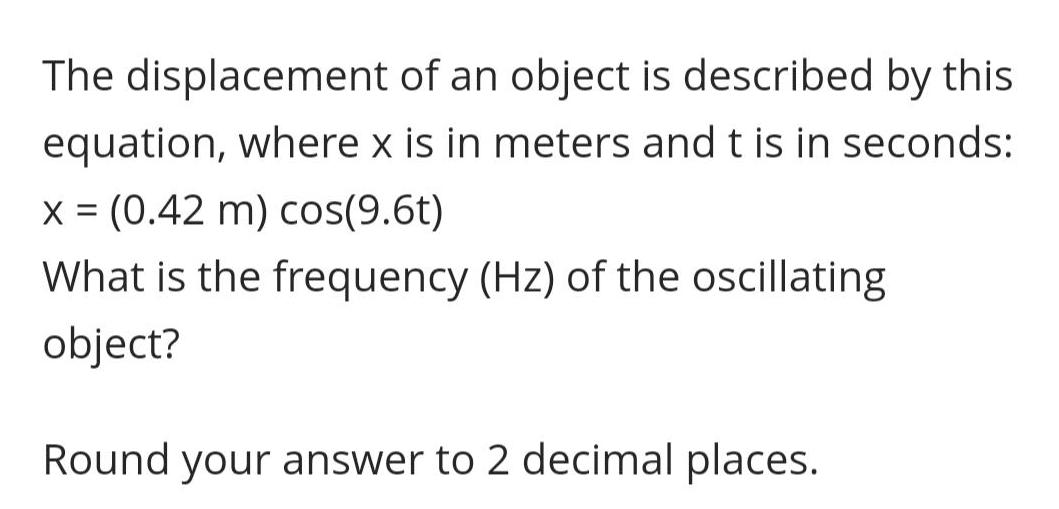Question:

# The displacement of an object is described by this equation,

Last updated: 8/7/2022The displacement of an object is described by this equation, where x is in meters and t is in seconds: x = (0.42 m) cos(9.6t) What is the frequency (Hz) of the oscillating object? Round your answer to 2 decimal places.